﻿ Analysis of Categorical Panel Data Scientific & Academic Publishing: The article detailed informationInternational Journal of Statistics and Applications

p-ISSN: 2168-5193    e-ISSN: 2168-5215

2012;  2(5): 56-59

doi: 10.5923/j.statistics.20120205.02

### Analysis of Categorical Panel Data

A. O. Adejumo , O. O. M. Sanni , E. T. Jolayemi , R. O. Ogedengbe

Department of Statistics University of Ilorin, Ilorin, Nigeria

Correspondence to: A. O. Adejumo , Department of Statistics University of Ilorin, Ilorin, Nigeria.
 Email: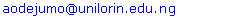Abstract

s In some categorical tables, one of the classifying variables may be at least ordinal (ranked) arising from a follow-up or any similar study. The other classifying variable(s) may be that which separates the population into groups using variables such as gender, race or location, or a combination of some of them. The counts obtained this way are analyzed recognizing that one of the variables is nearly metric and must be used and interpretation becomes easier when appropriate model is fitted to the arising product multinomial. An example of such an approach is provided using the data from Tuberculosis Management in a Teaching Hospital. We observed that the recovery rate of females was faster than their males counterpart on the assumption that those discharged through management system follows an exponential distribution.

Keywords: Panel data, Categorical data, Multinomial

Cite this paper: A. O. Adejumo , O. O. M. Sanni , E. T. Jolayemi , R. O. Ogedengbe , "Analysis of Categorical Panel Data", International Journal of Statistics and Applications, Vol. 2 No. 5, 2012, pp. 56-59. doi: 10.5923/j.statistics.20120205.02.

### 1. Introduction

Categorical data are obtained when the variables which are discrete in nature are cross-classified and subjects having the same levels of the cross-classification are aggregated to form counts. Clearly such variables are at most ordinal in nature. Variables that are purely metric are reduced appropriately for categorical data analysis to be effected. In a follow-up (longitudinal) study the progression of positive outcome is critical and should be examined.
Cross-classified data can have any of full-multinomial, hypergeometric, independent Poisson or product multinomial distributions, Bishop, Feinberg and Holland, Agresti, Sanni and Jolayemi, Adejumo among many authors. All these distributions have fixed, but unknown, parameters. Each underlying distribution is dictated by the sampling scheme, even though the parameter estimates within each are identical as demonstrated by Birch, see also Jolayemi et al. It is possible, however, that the parameters involved in the categorical data, have a specific pattern, especially when one or more of the categorical variables are metric but of constant interval. A statistical analysis approach for such data may be appropriate to use some models for probability outcomes. The model used, if appropriate can then be used to determine termination of management. This approach is in focus in this work.
In this research, the main objective is to examine a model fitting-algorithm for a longitudinal categorical data.
The follow-up data of this form becomes a panel data if the period for reassessment is constant.

### 2. Methodology

Consider an r x c contingency table. The row (r) categories are the sup-populations to be compared and column (c) equals the number of possible follow-ups. Let the matrix of observation be represented by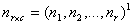,
where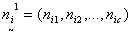(1)
Within the foregoing, assume the product multinomial distribution for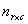. Thus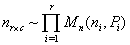(2)
where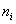is as represented in 1.1,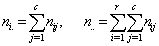and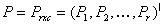such that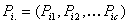and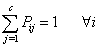(3)
Furthermore, assume that for each i, the vector Pi has a known or suspected pattern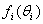. The mixture model is with a compelling assumption if eachis unique, see for example Brooks et al., when the variable characterizing the column is ordinal.
The main aim of this study is to test some hypothesis regarding. In particular, we assume thatis exponential in this research paper with parameters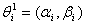In this formulation,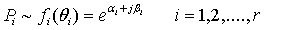(4)
where j=1,2, …, c; indicating the outcome of the column variable. If βi < 0, the probability reduces over j (usually indexing time) or over jth follow-up time of constant period. What may be of interest here are various hypotheses regarding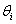. Some of these include.
(i)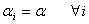which represents all r rows are identical before follow-up
(ii)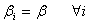which can be interpreted to be identical reactions of the r subpopulation for the intervention of the follow-up.
(iii)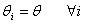is the combination of (i) and (ii) above.
Note that other forms ofare possible. Such other forms includes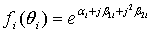which is essentially used when the response is quadratic. It is also used for studying medical intervention.
Let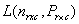be the likelihood function for.
Then,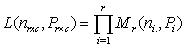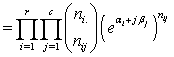so that the log likelihood L under the constraint in equation (1) is given by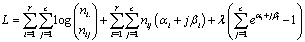(5)
where λ is the Langrange multiplier (indicating the boundary limit). Clearly the log likelihood of equation (5) does not give normal equations which are linear in the parameters, see McCulagh and Nelder, Jolayemi and Okoro for example.
Let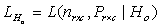be the likelihood function using estimation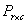under the null hypothesis and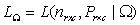be the similar likelihood under the parameter space.
The Likelihood ratio test statistic can be obtained from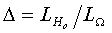Under some regularity conditions, see Bickel et al. and Adejumo,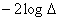has the chi-square distribution with (k-m) degrees of freedom, where k and m are the number of parameters estimated under Ω and Ho respectively.

#### 2.1. Estimation of Parameters

First consider the log likelihood function of equation (5) and let the null hypothesis Ho be given by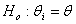This is equivalent to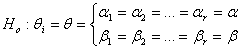which represents gender insensitivity. Other forms of Ho can be used.
The likelihood function LHo is given by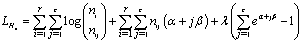(6)
The normal equations from equation (6) are obtained as follows: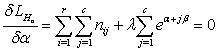(7)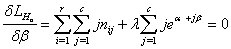(8)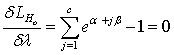(9)
From equation (7) it is clear that λ = -n..
Thus equations (7) and (8) become respectively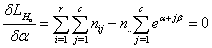(10)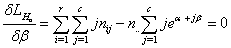(11)
Let S2x1 represent the vector of normal equations.
Then S is given by
So that the Hessian matrix, Morisson is given by(12)
Let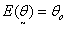, then by mid-value theorem: Mood et al.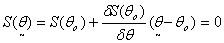(13)
so that the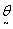can be obtained as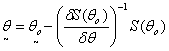An iterative procedure is then used to obtain an estimate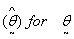using an initial vector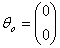and tolerance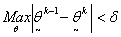(14)
It is easy to note that the vector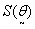is given by
And the cell values of the Hessian matrix is given by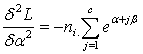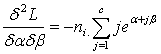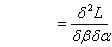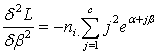Under Ω, the above procedure is obtained for each i.
Thus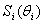Finally, the estimate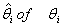is obtained as explained earlier. The test statistic in this case which is the likelihood ratio test statistic is given by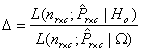So thatwhere d = (k - m) degrees of freedom. (k - m) = (2r - 2)=2(r - 1).
If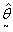under Ho is given as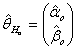And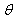under Ω is given as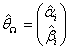Then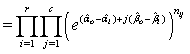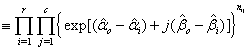And -2log∆ is given as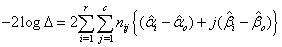The above is a demonstration of how to produce software to perform the process for execution.

### 3. Empirical Results, Discussions and Conclusions

The method of application of mixture models for the 2-dimensional categorical data is demonstrated using a data set from a disease management from a hospital, the University of Ilorin Teaching Hospital (UITH), Nigeria, spanning the period between 1996 and 1998 on the management of Tuberculosis patients. The data excluded those who were lost to follow-up, so that, those who were successfully discharged were considered in Table 1 using approximated periods.
 Table 1. 109 Tuberculosis patients classified by length of treatment and gender using one month follow up interval
 Duration (in month) 1 2 3 4 5 6 Total No. of Male 44 17 6 3 3 1 74 No. of Female 22 8 4 1 0 0 35 Total 66 25 10 4 3 1 109
The analysis of the data followed equation (2) and the imposed models in equation (4). Using the tolerance limit δ = 0.001 for maximum difference in the parameter estimates as dictated by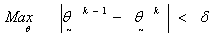of equation (13), the following estimates were obtained: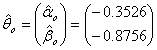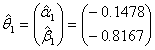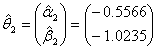The likelihood ratio test statistic for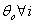of G2=-2log∆=15.24 with d=2 degrees of freedom with p-value of 0.001 provided a bad fit
This implies that a uniform distribution cannot be used for both males and females. Consequently, different models existed for males and females which were θ1 and θ2. This showed that the period of treatment was gender sensitive. While males would be treated for seven months the female counterpart would be treated for 4 months.

### References

  Bishop, Y. M. M, Feinberg, S. E. Holland, P. W. (1975). Discrete Multivariate Analysis. Cambridge MA; MIT Press.  Agresti, A. (2002). Categorical Data Analysis. John Wiley and Sons. 2nd Edition. New York.  Sanni, O. O. M. and Jolayemi, E. T. (1998). On the use of some Categorical Test Statistic on Sparse Contingency Table. Journal of Pure and Applied Science. 509 – 514.  Adejumo, A. O (2005). Modelling Generalized Linear (Loglinear) Models for Raters Agreement measures; Peter Lang, Frankfurt am Main. (http://www.peterlang.de)  Birch, M. W. (1966). Maximum Likelihood in Three Way contingency Table. J. Royal Statistics Society, Series B25, 220 – 233.  Jolayemi, E. T. and Brown, M. B. (1984). The Choice of a log-linear model using Cp-type Statistics. Computational Statistics and Data Analysis.  Brooks, S. P, Morgan, B. J. T, Riobut, M. S, and Peak, S.C. (1997). Finite Mixture Models for Proportions. Biometric, 53; 1097 – 1115.  McCullagh, P. and Nelder, J. A. (1989). Generalised Linear Models. Chapman and Hall. New York.  Jolayemi, E. T. and Okoro, E. O (1995). On the estimation of mean IC50. Biosciences Research Communication, 7, 175 – 178.  Bickel, P. J. and Doksum, J. A. (1973). Mathematical Statistics. Holden Day, San Francisco.  Morrison, D. (1976). Multivariate Statistics Methods. McGraw Hill, New York.  Mood, A. M., Graybill, F. A., and Boes, D. C. (1963). Introduction to the Theory of Statistics. McGraw Hill, New York.